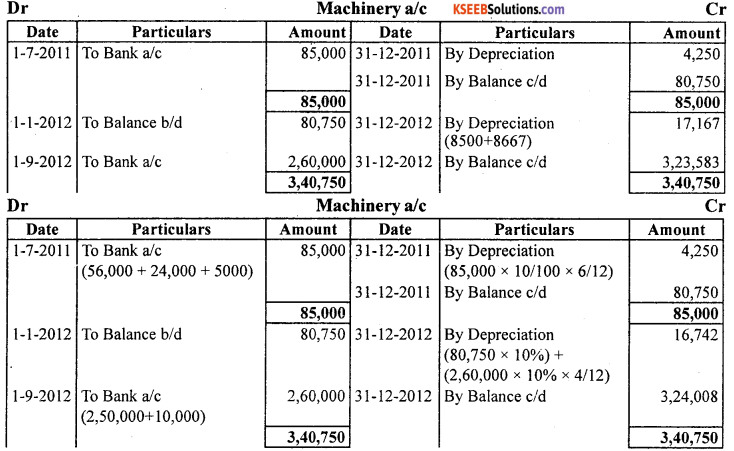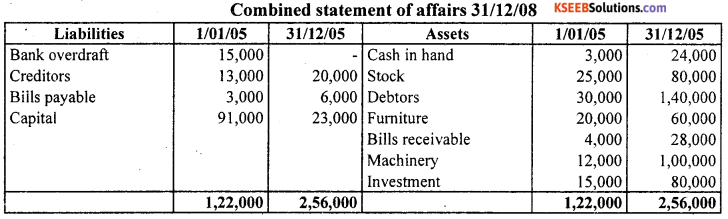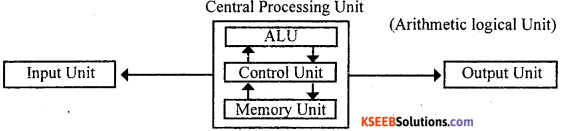# 1st PUC Accountancy Question Bank Practical Oriented Questions

## Karnataka 1st PUC Accountancy Question Bank Practical Oriented Questions

### 1st PUC Practical Oriented Questions

Question 1.
Mention the accounting equation and also give examples with balance sheet.
Accounting equation => Capital = Assets + Liability
Example:
(i) Anil started business with cash Rs. 50000 Accounting equation => Capital = Assets + Liabilities
∴ Capital = 50,000, Assets (cash) = 50,000
∴ 50,000 =50,000

(ii) Purchase goods for Rs. 20,000
Capital = 50,000, Cash = 50,000-20,000 = 30,000 Stock = 2,000
∴ Capital = Cash + Stock
50,000 = 30,000 + 20,000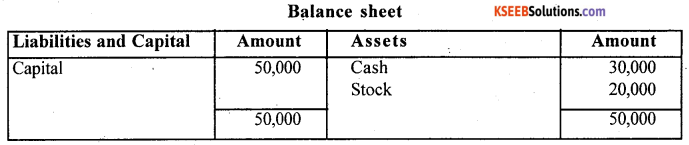Question 2.
Write the specimen of accounting cycle.2009, 2011(S), 2008, 2009, 2010(N), 2012(N)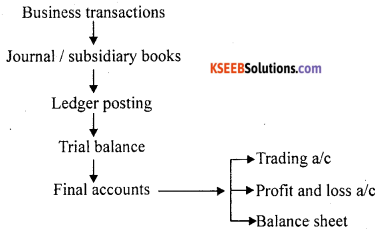Question 3.
Pass journal entries from the ledger given. 2009(S)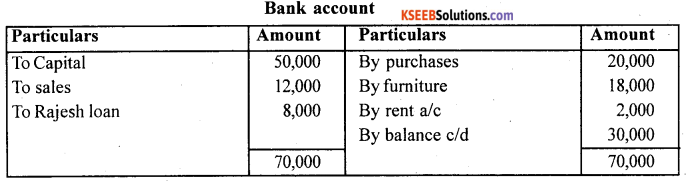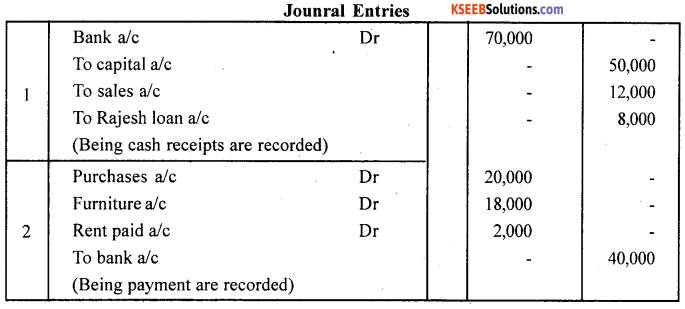Question 4.
Prepare the format of a bills of exchange. 2008(N), 2009, 2010. 2011(S), 2012(N) and (S)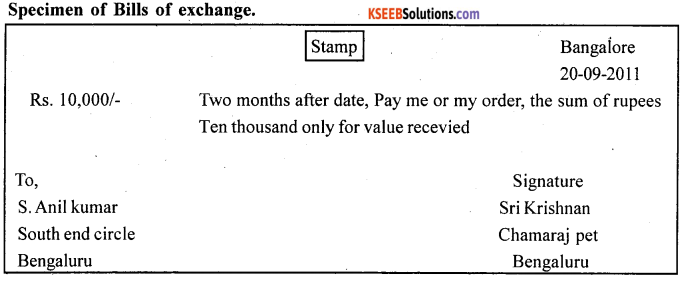Question 5.
Give the speciman of an Invoice. 2011(S), 20011(N)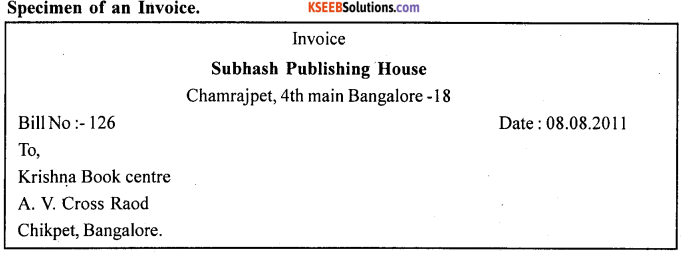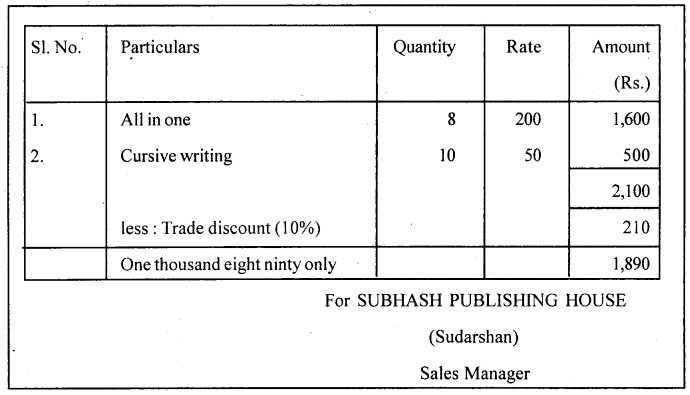Question 6.
Write the speciman of debit note. 2009, 2010(N) 2009(S)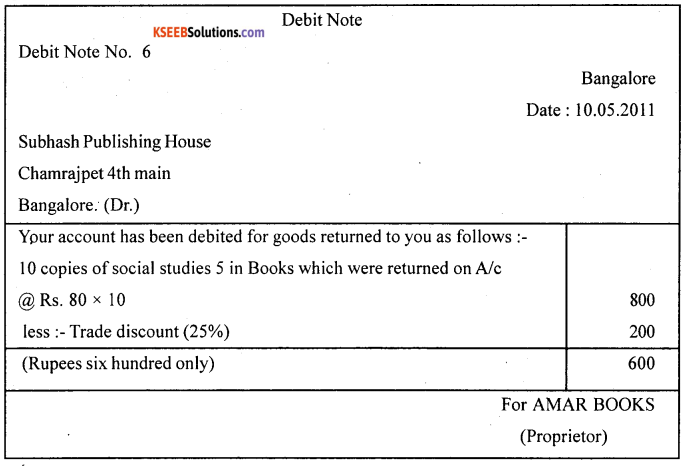Question 7.
Write the speciman of credit note.
Specimen of Credit note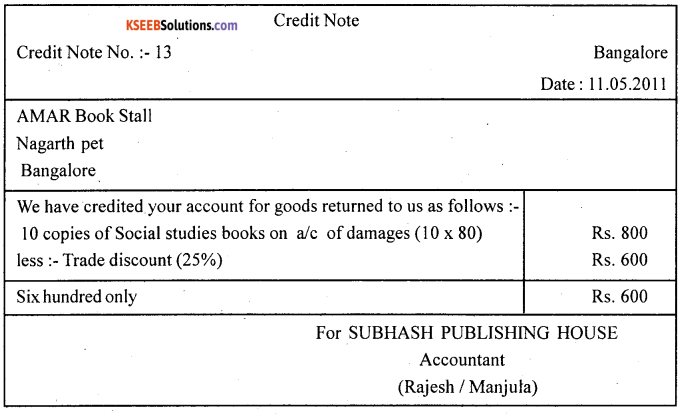Question 8.
Write the accounting equation find out missing figures. 2009(N)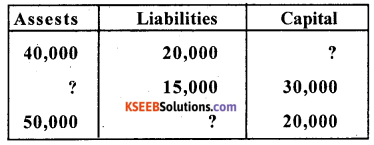Accounting equation
Asset = Capital + Liabilities
(i) 40,000 = Capital-20,000
Capital = 40,000 -20,000
Capital = 20,000

(ii) Assets = 15,000 + Liabilities + 20,000
∴ Assets = 45,000

(iii) 50,000 = Liabilities + 2,000
Liabilities = 50,000 – 20,000
∴ Liabilities = 30,000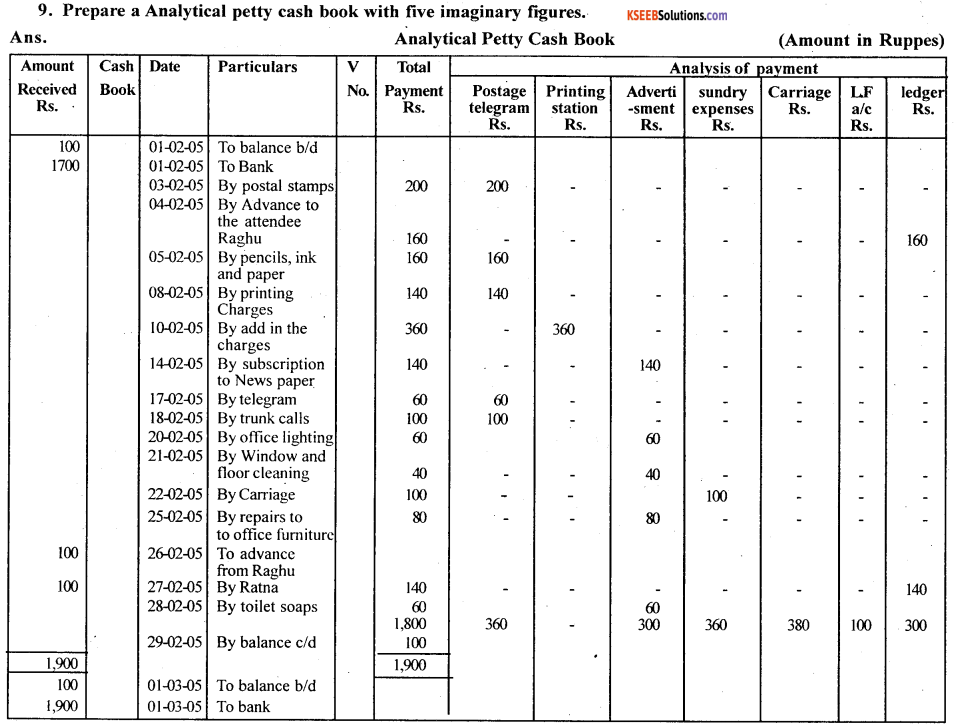Question 10.
Prepare a trading a/c with five imaginary figures. 2008(N), 2010(S), 2012(N)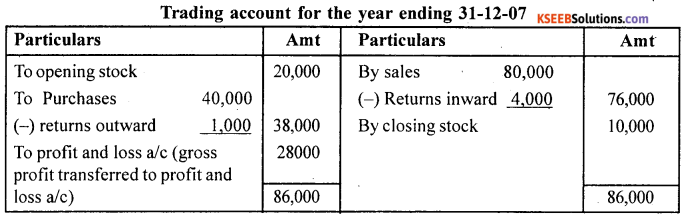Question 11.
Write the specimen of profit and loss account with imaginary figures.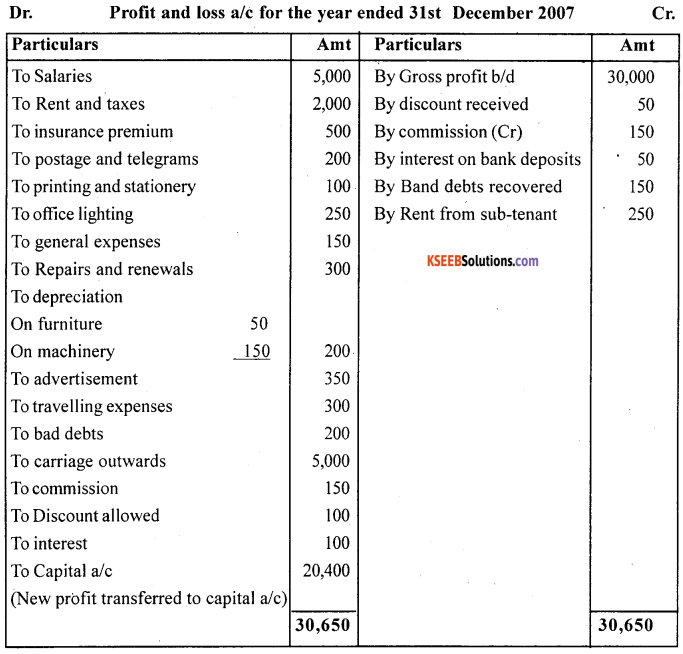Question 12.
Prepare Balance sheet speciman with imaginary figures. 2010(S)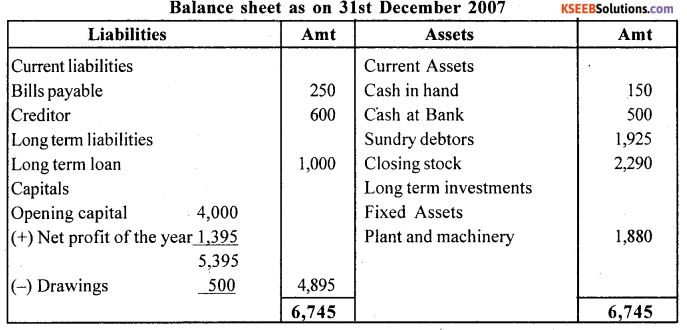Question 13.
Prepare a Trial balance with ten imaginary figures. 2008(S)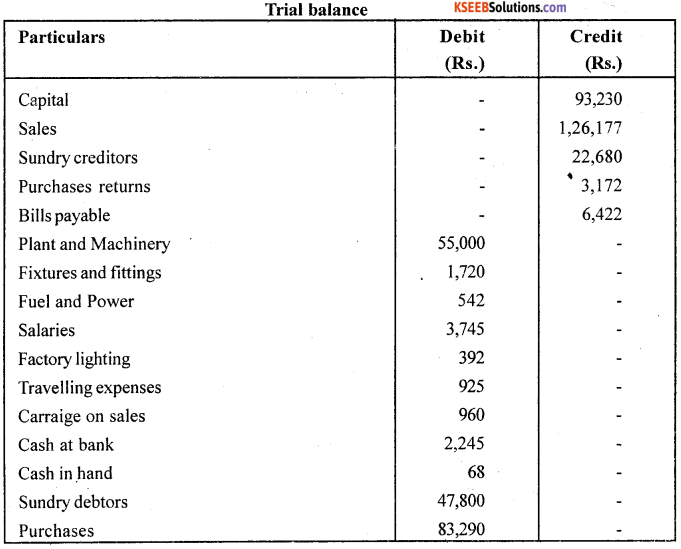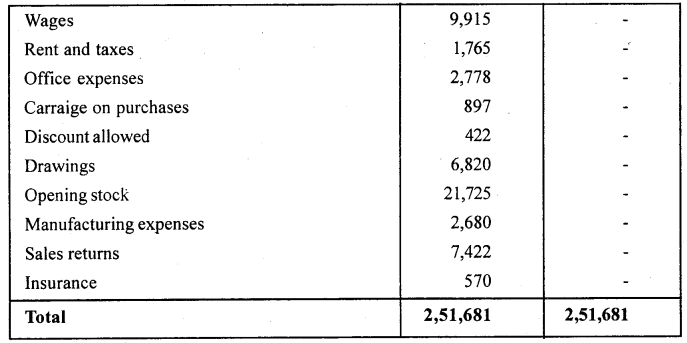Question 14.
Prepare a capital ledger a/c for the following transaction.
01- 07 – 2008 Savith commenced business with cash Rs. 20000 machinery Rs. 10000 and building Rs. 15000.
18 – 07 – 2008 She withdraw Rs. 5000
20 – 07 – 2008 the netprofit earned rs. 1,000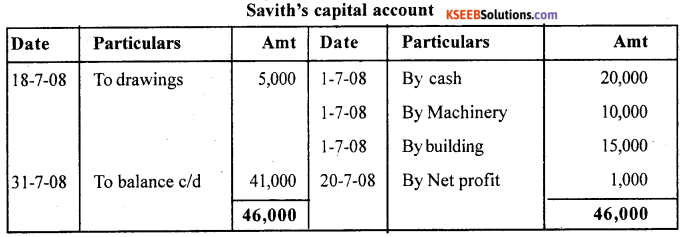Question 15.
Write the specimen of journal and ledger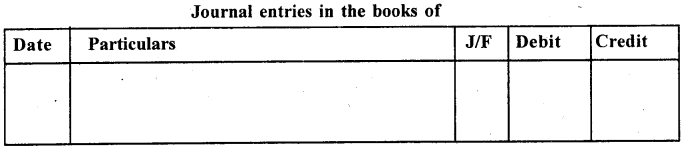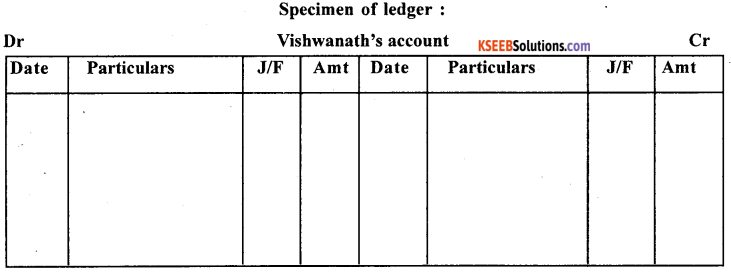Question 16.
Write the specimen of simple cash book with five imaginary items. 2010(N), 2008, 2010(S)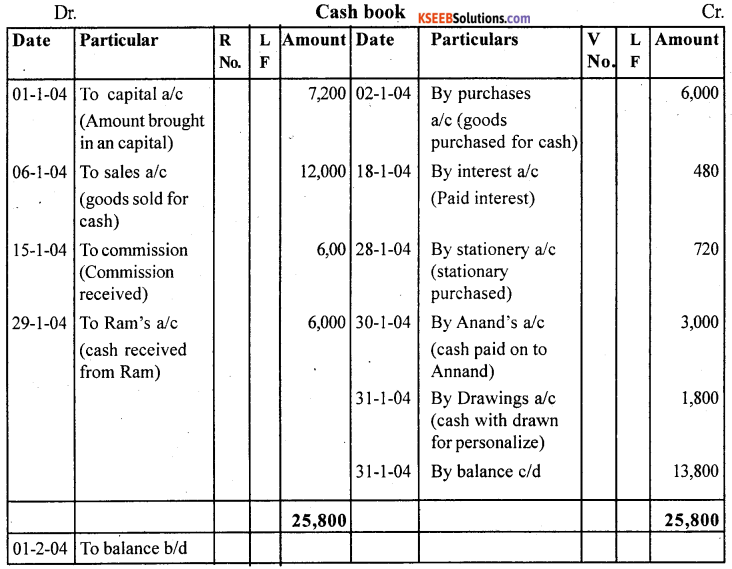Question 17.
Prepare a machinery account for two years with imaginary figures under
(a) Straight line method
(b) Diminishing balance method.